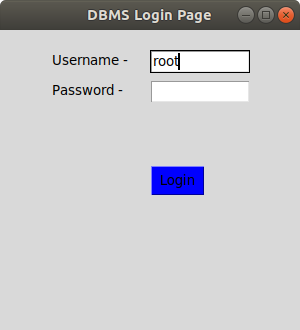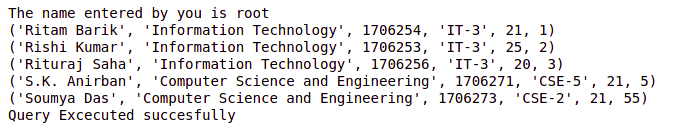Related Articles

# Create MySQL Database Login Page in Python using Tkinter

• Last Updated : 27 Aug, 2021

Prerequisites: Python GUI – tkinter, Python MySQL – Select Query
Tkinter is one of the Python libraries which contains many functions for the development of graphic user interface pages and windows. Login pages are important for the development of any kind of mobile or web application. This page is most essential for user authentication purposes.
We will use mysql.connector library to establish a connection between Python project and MySQL workbench. Db is the object created using mysql.connector.connect class which stores all the information about databases such database name, password, and table name.

In the below example,

• tk.label and tk.button is used to create labels and buttons on the GUI screen. Every button contains a command in it which includes a function to be executed on click of the button.
• The function logintodb is created to login into the MySQL Database. The save query includes the query to be executed on the click of the submit button.
• X and Y are the parameters given to adjust objects on the Tkinter window.
• Root.mainloop() is included at the last indicating that only components within it are included in the window.

Below is the implementation:

## Python3

 `import` `tkinter as tk``import` `mysql.connector``from` `tkinter ``import` `*`` `  `def` `submitact():``    ` `    ``user ``=` `Username.get()``    ``passw ``=` `password.get()`` ` `    ``print``(f``"The name entered by you is {user} {passw}"``)`` ` `    ``logintodb(user, passw)`` `  `def` `logintodb(user, passw):``    ` `    ``# If password is enetered by the``    ``# user``    ``if` `passw:``        ``db ``=` `mysql.connector.connect(host ``=``"localhost"``,``                                     ``user ``=` `user,``                                     ``password ``=` `passw,``                                     ``db ``=``"College"``)``        ``cursor ``=` `db.cursor()``        ` `    ``# If no password is enetered by the``    ``# user``    ``else``:``        ``db ``=` `mysql.connector.connect(host ``=``"localhost"``,``                                     ``user ``=` `user,``                                     ``db ``=``"College"``)``        ``cursor ``=` `db.cursor()``        ` `    ``# A Table in the database``    ``savequery ``=` `"select * from STUDENT"``    ` `    ``try``:``        ``cursor.execute(savequery)``        ``myresult ``=` `cursor.fetchall()``        ` `        ``# Printing the result of the``        ``# query``        ``for` `x ``in` `myresult:``            ``print``(x)``        ``print``(``"Query Excecuted successfully"``)``        ` `    ``except``:``        ``db.rollback()``        ``print``(``"Error occured"``)`` `  `root ``=` `tk.Tk()``root.geometry(``"300x300"``)``root.title(``"DBMS Login Page"``)`` `  `# Defining the first row``lblfrstrow ``=` `tk.Label(root, text ``=``"Username -"``, )``lblfrstrow.place(x ``=` `50``, y ``=` `20``)` `Username ``=` `tk.Entry(root, width ``=` `35``)``Username.place(x ``=` `150``, y ``=` `20``, width ``=` `100``)`` ` `lblsecrow ``=` `tk.Label(root, text ``=``"Password -"``)``lblsecrow.place(x ``=` `50``, y ``=` `50``)` `password ``=` `tk.Entry(root, width ``=` `35``)``password.place(x ``=` `150``, y ``=` `50``, width ``=` `100``)` `submitbtn ``=` `tk.Button(root, text ``=``"Login"``,``                      ``bg ``=``'blue'``, command ``=` `submitact)``submitbtn.place(x ``=` `150``, y ``=` `135``, width ``=` `55``)` `root.mainloop()`

Output:Attention geek! Strengthen your foundations with the Python Programming Foundation Course and learn the basics.

To begin with, your interview preparations Enhance your Data Structures concepts with the Python DS Course. And to begin with your Machine Learning Journey, join the Machine Learning – Basic Level Course

My Personal Notes arrow_drop_up